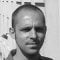Learn about power engineering and HV/MV/LV substations. Study specialized technical articles, electrical guides, and papers.

# Ohm’s Law – The Resistance

Home / Technical Articles / Ohm’s Law – The ResistanceIt is intuitive that voltage and current would be somehow related. For example, if the potential difference between two ends of a wire is increased, we would expect a greater current to flow, just like the flow rate of gas through a pipeline increases when a greater pressure difference is applied.

For most materials, including metallic conductors, this relationship between voltage and current is linear: as the potential difference between the two ends of the conductor increases, the current through the conductor increases proportionally.

This statement is expressed in Ohm’s law:

## V = I R

To say that Ohm’s law is true for a particular conductor is to say that the resistance of this conductor is, in fact, constant with respect to current and voltage. Certain materials and electronic devices exhibit a nonlinear relationship between current and voltage, that is, their resistance varies depending on the voltage applied.

The relationship V = IR will still hold at any given time, but the value of R will be a different one for different values of  V and I.

These nonlinear devices have specialized applications and will not be discussed in this article. Resistance also tends to vary with temperature, though a conductor can still obey Ohm’s law at any one temperature.

For example, the resistance of a copper wire increases as it heats up. In most operating regimes, these variations are negligible. Generally, in any situation where changes in resistance are significant, this is explicitly mentioned. Thus, whenever one encounters the term “resistance” without further elaboration, it is safe to assume that within the given context, this resistance is a fixed, unchanging property of the object in question.

Resistance depends on an object’s material composition as well as its shape. For a wire, resistance increases with length, and decreases with cross-sectional area.

Again, the analogy to a gas or water pipe is handy: we know that a pipe will allow a higher flow rate for the same pressure difference if it has a greater diameter, while the flow rate will decrease with the length of the pipe. This is due to friction in the pipe, and in fact, an analogous “friction” occurs when an electric current travels through a material.

This friction can be explained by referring to the microscopic movement of electrons or ions, and noting that they interact or collide with other particles in the material as they go. The resulting forces tend to impede the movement of the charge carriers and in effect limit the rate at which they pass. These forces vary for different materials because of the different spatial arrangements of electrons and nuclei, and they determine the material’s ability to conduct.

This intrinsic material property, independent of size or shape, is called resistivity and is denoted by ρ (the Greek lowercase rho).

The actual resistance of an object is given by the resistivity multiplied by the length of the object (l) and divided by its cross-sectional area (A):The units of resistance are ohms, abbreviated Ω (Greek capital omega). By rearranging Ohm’s law, we see that resistance equals voltage divided by current. Units of resistance are thus equivalent to units of voltage divided by units of current. By definition, one ohm equals one volt per ampere (Ω = V/A).

The units of resistivity are ohm-meters (Ω-m), which can be reconstructed through the preceding formula: when ohm-meters are multiplied by meters (for l) and divided by square meters (for A), the result is simply ohms. Resistivity, which is an intrinsic property of a material, is not to be confused with the resistance per unit length (usually of a wire), quoted in units of ohms per meter (Ω/m).

The latter measure already takes into account the wire diameter; it represents, in effect, the quantity ρ/A. The resistivities of different materials in Ω-m can be found in engineering tables.

SOURCE: Electric Power Systems – A. Von Meir

Get access to premium HV/MV/LV technical articles, electrical engineering guides, research studies and much more! It helps you to shape up your technical skills in your everyday life as an electrical engineer.### Edvard Csanyi

Electrical engineer, programmer and founder of EEP. Highly specialized for design of LV/MV switchgears and LV high power busbar trunking (<6300A) in power substations, commercial buildings and industry facilities. Professional in AutoCAD programming.

1.Ibrahim Seder
Nov 07, 2011

Thanks Edvard, I think semiconductors are the best examples that don’t obey Ohm’s Law.
BR

•Edvard
Nov 07, 2011

Hehe, you’re right! Thanks Seder!

2.Odongo Elly Ampeire
Sep 02, 2011

Hello .

I appreicate the work that you are doing, and i wpuld like to recieve added infprmation and literature in regarding to the above information .

Elly

•eng.eslam
Sep 02, 2011

thanks very much